## Wednesday, July 15, 2009

### Hurwitz zeta function, Dirichlet L-series, Appell F1

I've added three more functions to mpmath since the last blog update: the Hurwitz zeta function, Dirichlet L-series and the Appell hypergeometric function.

#### Hurwitz zeta function

The Hurwitz zeta function is available with the syntax hurwitz(s, a=1, derivative=0). It's a separate function from zeta for various reasons (one being to make the plain zeta function as simple as possible). With the optional third argument, it not only computes values, but arbitrarily high derivatives with respect to s (more on which later).

It's quite fast at high precision; e.g. the following evaluation takes just 0.25 seconds (0.6 seconds from cold cache) on my laptop:

`>>> mp.dps = 1000>>> hurwitz(3, (1,3))        # (1,3) specifies the exact parameter 1/327.56106119970080377622787797740750928454209531301488108286446031855695818017193319714478144925225572428339288398963479601630790061026511179978568818123994067840573189334947537240910125121134668254531419227282374538466526604902207819804397999746337871597509019667252833751410875882452970087673701166818534638337151352084773537656137422271798879545153523879812143981699853880764694094326889123555060920043334937078540793636560719603896550074378037796559947176109732627192261674381230503180321801486524844073873393780084553765789276561564723000726671702263215399293512078780185479463617569113965545079189333448241385996978516660755944879572783482345047875282167516302446341564720560895973910007102633691282693068326115586706265136825191025873785920661492516925137079914816949085911457084632783280741306988974583447464692728282674849897565952599172908008487680740297664177868157329190791734974760032062614100444842998745085439117586813879301569134308141720962503488644344900903488585429308918210445984048`

With s = π instead, it takes 3.5 seconds. For comparison, Mathematica 6 took 0.5 and 8.3 seconds respectively (on an unloaded remote system which is faster than my laptop, but I'm not going to guess by how much).

The function is a bit slow at low precision, relatively speaking, but fast enough (in the 1-10 millisecond range) for plotting and such. Of course, it works for complex s, and in particular on the critical line 1/2+it. Below, I've plotted |ζ(1/2+it,1)|2, |ζ(1/2+it,1/2)|2, |ζ(1/2+it,1/3)|2; the first image shows 0 ≤ t < 30 and the second is with 1000 ≤ t < 1030.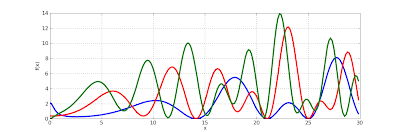To show some more complex values, here are plots of ζ(s, 1), ζ(s, 1/3) and ζ(s, 24/25) for 100 ≤ Im(s) ≤ 110. I picked the range [-2, 3] for the real part to show that the reflection formula for Re(s) < 0 works:In fact, the Hurwitz zeta implementation works for complex values of both s and a. Here is a plot of hurwitz(3+4j, a) with respect to a:The evaluation can be slow and/or inaccurate for nonrational values of a, though (in nonconvergent cases where the functional equation isn't used).

#### Zeta function performance

Right now the implementations of the Riemann zeta function and the Hurwitz zeta function in mpmath are entirely separate. In fact, they even use entirely different algorithms (hurwitz uses Euler-Maclaurin summation, while zeta uses the Borwein approximation). This is useful for verifying correctness of either function.

Regarding performance, it appears that the Borwein approximation is slightly faster for small |Im(s)| while Euler-Maclaurin is massively faster for large |s|.

The following benchmark might give an idea:

`>>> from mpmath import *>>> mp.dps = 15; mp.pretty = True>>> timing(zeta, 0.5+10j); timing(hurwitz, 0.5+10j)0.00273861622077666270.0082901877193889192>>> timing(zeta, 0.5+100j); timing(hurwitz, 0.5+100j)0.00753193492469010890.041037198861866478>>> timing(zeta, 0.5+1000j); timing(hurwitz, 0.5+1000j)0.134684994880634790.18062463246027072>>> timing(zeta, 0.5+10000j); timing(hurwitz, 0.5+10000j)7.29775935209642411.0488757649366072>>> timing(zeta, 0.5+10000j); timing(hurwitz, 0.5+10000j)0.843066367373509711.0601629536714867`

As the last evaluation shows, the Borwein algorithm is still not doing too poorly at 0.5+10000j from a warm cache, but at this point the cache is 50 MB large. At 0.5+100000j my laptop ran out of RAM and Firefox crashed (fortunately Blogger autosaves!) so it clearly doesn't scale. The Euler-Maclaurin algorithm caches a lot of Bernoulli numbers, but the memory consumption of this appears to be negligible. With hurwitz (and the function value, for those interested),

`>>> timing(hurwitz, 0.5+100000j)8.0361576680835149>>> hurwitz(0.5+100000j)(1.07303201485775 + 5.7808485443635j)`

and the memory usage of the Python interpreter process only climbs to 12 MB.

Just by optimizing the pure Python code, I think the Hurwitz zeta function (at low precision) could be improved by about a factor 2 generally and perhaps by a factor 3-4 on the critical line (where square roots can be used instead of logarithms -- zeta uses this optimization but hurwitz doesn't yet); with Cython, perhaps by as much as a factor 10.

The real way to performance is not to use arbitrary-precision arithmetic, though. Euler-Maclaurin summation for zeta functions is remarkably stable in fixed-precision arithmetic, so there is no problem using doubles for most applications. As I wrote a while back on sage-devel, a preliminary version of my Hurwitz zeta code for Python complex was 5x faster than Sage's CDF zeta (in a single test, mind you). If there is interest, I could add such a version, perhaps writing it in Cython for Sage (for even greater speed).

#### Derivatives

The Hurwitz zeta function can be differentiated easily like so:

`>>> mp.dps = 25>>> hurwitz(2+3j, 1.25, 3)(-0.01266157985314340398338543 - 0.06298953579777517606036907j)>>> diff(lambda s: hurwitz(s, 1.25), 2+3j, 3)(-0.01266157985314340398338543 - 0.06298953579777517606036907j)`

For simple applications one can just as well use the numerical diff function as above with reasonable performance, but this isn't really feasible at high precision and/or high orders (numerically computing an nth derivative at precision p requires n+1 function evaluations, each at precision (n+1)×p).

The specialized code in hurwitz requires no extra precision (although it still needs to perform extra work) and therefore scales up to very high precision and high orders. Here for example is a derivative of order 100 (taking 0.5 seconds) and one of order 200 (taking 4 seconds):

`>>> hurwitz(2+3j, 1.25, 100)(2.604086240825183469410664e+107 - 1.388710675207202633247271e+107j)>>> hurwitz(2+3j, 1.25, 200)(2.404124816484789309285653e+274 + 6.633220818921777955999455e+273j)`

It should be possible to calculate a sequence of derivatives much more quickly than with separate calls, although this isn't implemented yet. One use for this is to produce high-degree Taylor series. This could potentially be used in a future version of mpmath, for example to provide very fast moderate-precision (up to 50 digits, say) function for multi-evaluation of the Riemann zeta function on the real line or not too far away from the real line. This in turn would speed up other functions, such as the prime zeta function and perhaps polylogarithms in some cases, which are computed using series over many Riemann zeta values.

#### Dirichlet L-series

With the Hurwitz zeta function in place, it was a piece of cake to also supply an evaluation routine for arbitrary Dirichlet L-series.

The way it works it that you provide the character explicitly as a list (so it also works for other periodic sequences than Dirichlet characters), and it evaluates it as a sum of Hurwitz zeta values.

For example (copypasting from the docstring), the following defines and verifies some values of the Dirichlet beta function, which is defined by a Dirichlet character modulo 4:

`>>> B = lambda s, d=0: dirichlet(s, [0, 1, 0, -1], d)>>> B(0); 1./20.50.5>>> B(1); pi/40.78539816339744830961566090.7853981633974483096156609>>> B(2); +catalan0.91596559417721901505460350.9159655941772190150546035>>> B(2,1); diff(B, 2)0.081580736116592795102912170.08158073611659279510291217>>> B(-1,1); 2*catalan/pi0.58312180806163756027676890.5831218080616375602767689>>> B(0,1); log(gamma(0.25)**2/(2*pi*sqrt(2)))0.39159439270683677647194530.3915943927068367764719454>>> B(1,1); 0.25*pi*(euler+2*ln2+3*ln(pi)-4*ln(gamma(0.25)))0.19290131679691242936318980.1929013167969124293631898`

#### Appell hypergeometric function

The function appellf1 computes the Appell F1 function which is a hypergeometric series in two variables. I made the implementation reasonably fast by rewriting it as a single series in hyp2f1 (with the side effect of supporting the analytic continuation with that for 2F1).

A useful feature of the Appell F1 function is that it provides a closed form for some integrals, including those of the form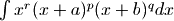with arbitrary parameter values, for arbitrary endpoints (and that's a quite general integral indeed). Comparing with numerical quadrature:

`>>> def integral(a,b,p,q,r,x1,x2):...     a,b,p,q,r,x1,x2 = map(mpmathify, [a,b,p,q,r,x1,x2])...     f = lambda x: x**r * (x+a)**p * (x+b)**q...     def F(x):...         v = x**(r+1)/(r+1) * (a+x)**p * (b+x)**q...         v *= (1+x/a)**(-p)...         v *= (1+x/b)**(-q)...         v *= appellf1(r+1,-p,-q,2+r,-x/a,-x/b)...         return v...     print "Num. quad:", quad(f, [x1,x2])...     print "Appell F1:", F(x2)-F(x1)...>>> integral('1/5','4/3','-2','3','1/2',0,1)Num. quad: 9.073335358785776206576981Appell F1: 9.073335358785776206576981>>> integral('3/2','4/3','-2','3','1/2',0,1)Num. quad: 1.092829171999626454344678Appell F1: 1.092829171999626454344678>>> integral('3/2','4/3','-2','3','1/2',12,25)Num. quad: 1106.323225040235116498927Appell F1: 1106.323225040235116498927`

Also incomplete elliptic integrals are covered, so you can for example define one like this:

`>>> def E(z, m):...     if (pi/2).ae(z):...         return ellipe(m)...     return 2*round(re(z)/pi)*ellipe(m) + mpf(-1)**round(re(z)/pi)*\...         sin(z)*appellf1(0.5,0.5,-0.5,1.5,sin(z)**2,m*sin(z)**2)...>>> z, m = 1, 0.5>>> E(z,m); quad(lambda t: sqrt(1-m*sin(t)**2), [0,pi/4,3*pi/4,z])0.92732988362444006696590420.9273298836244400669659042>>> z, m = 3, 2>>> E(z,m); quad(lambda t: sqrt(1-m*sin(t)**2), [0,pi/4,3*pi/4,z])(1.057495752337234229715836 + 1.198140234735592207439922j)(1.057495752337234229715836 + 1.198140234735592207439922j)`

The Appell series isn't really faster than numerical quadrature, but it's possibly more robust (the singular points need to be given to quad as above to obtain any accuracy, for example). Mpmath doesn't yet have incomplete elliptic integrals; the version above could be a start, although I'll probably want to try a more canonical approach.

## Sunday, July 12, 2009

### Another Mathematica bug

Mathematica is great for cross-checking numerical values, but it's not unusual to run into bugs, so triple checking is a good habit.

Here is mpmath's evaluation for two Struve function values:

`>>> struvel(1+j, 100j)(0.1745249349140313153158106 + 0.08029354364282519308755724j)>>> struvel(1+j, 700j)(-0.1721150049480079451246076 + 0.1240770953126831093464055j)`

The same values in Mathematica:

`In:= N[StruveL[1+I, 100I], 25]Out= 0.1745249349140313153158107 + 0.0802935436428251930875572 IIn:= N[StruveL[1+I, 700I], 25]Out= -0.2056171312291138282112197 + 0.0509264284065420772723951 I`

I'm almost certain that the second value returned by Mathematica is wrong. The value from mpmath agrees with a high-precision direct summation of the series defining the Struve L function, and even Mathematica gives the expected value if one rewrites the L function in terms of the H function:

`In:= n=1+I; z=700IOut= 700 IIn:= N[-I Exp[-n Pi I/2] StruveH[n, I z], 25]Out= -0.1721150049480079451246076 + 0.1240770953126831093464055 I`

Maple also agrees with mpmath:
`> evalf(StruveL(1+I, 700*I), 25);        -0.1721150049480079451246076 + 0.1240770953126831093464055 I`

So unless Mathematica uses some nonstandard definition of Struve functions, unannounced, this very much looks like a bug in their implementation.

Wolfram Alpha reproduces the faulty value, so this still appears to be broken in Mathematica 7.

## Friday, July 10, 2009

### Improved incomplete gamma and exponential integrals; Clausen functions

The SVN trunk of mpmath now contains much improved implementations of the incomplete gamma function (gammainc()) as well as the exponential integrals (ei(), e1(), expint()). Although the code is not quite perfect yet, this was a rather tedious undertaking, so I'm probably going to work on something entirely different for a while and give these functions another iteration later.

The incomplete gamma function comes in three flavors: the lower incomplete gamma function, the upper incomplete gamma function, and the generalized (two-endpoints) incomplete gamma function. The generalized incomplete gamma function is defined aswhich reduces to the lower function when a = 0, and the upper version when b = +∞. A huge number of integrals occurring in pure and applied mathematics have this form (even Gaussian integrals, with a change of variables) so a solid incomplete gamma is quite important. It's especially important to ensure both speed in correctness in asymptotic cases.

The lower incomplete gamma function is easiest to implement, because it's essentially just a rescaled version of the hypergeometric 1F1 function and the 1F1 implementation already works well. Not much change was made here, so I'm going to write some about the other cases instead.

The exponential integrals are essentially the same as the upper incomplete gamma function and mostly share code. Remarks about the upper gamma function therefore also apply to the exponential integrals.

#### Upper gamma performance

The upper incomplete gamma function is hard because the two main series representations are an asymptotic series involving 2F0 that doesn't always converge, and an 1F1 series that suffers badly from cancellation for even moderately large arguments. The problem is to decide when to use which series.

The 2F0 series is very fast when it converges, while the 1F1 series is quite slow (due to the need for extra precision) just below the point where 2F0 starts to converge. After some experimentation, I decided to change the implementation of 2F0. Instead of performing a heuristic, conservative test to determine whether the series will converge (sometimes claiming falsely that it won't), it now always goes ahead to sum the series and raises an error only when it actually doesn't converge.

Thus the asymptotic series will always be used when possible, and although this leads to a slight slowdown for smaller arguments, it avoids worst-case slowness. The most important use for the incomplete gamma function, I believe, is in asymptotics, so I think this is a correct priority.

As a result, you can now do this:

`>>> from mpmath import *>>> mp.dps = 25; mp.pretty = True>>> gammainc(10, 100)4.083660630910611272288592e-26>>> gammainc(10, 10000000000000000)5.290402449901174752972486e-4342944819032375>>> gammainc(3+4j, 1000000+1000000j)(-1.257913707524362408877881e-434284 + 2.556691003883483531962095e-434284j)`

The following graph compares old and new performance. The y axis shows the reciprocal time (higher is better) for computing gammainc(3.5, x) as x ranges between 0 and 100, at the standard precision of 15 digits. Red is the old implementation and blue is the new. The code also works with complex numbers, of course; replacing x with j*x gives a virtually identical graph (slightly scaled down due to the general overhead of complex arithmetic).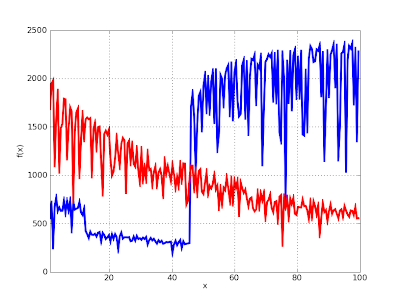It's very visible where the asymptotic series kicks in, and the speed from then on is about 2000 evaluations per second which is relatively good. The new implementation is regrettably up to 3x slower than the old one for smaller x, although the slowdown is a bit misleading since the old version was broken and gave inaccurate results. The big dip in the blue graph at x = 10 is due to the automatic cancellation correction which the old code didn't use.

The gap between the asymptotic and non-asymptotic cases could be closed by using specialized series code for the lower incomplete gamma function, or using the Legendre continued fraction for intermediate cases (this comes with some problems however, such as accurately estimating the rate of convergence, and the higher overhead for evaluating a continued fraction than a series). This will certainly be worth doing, but I'm not going to pursue those optimizations right now for reasons already stated.

Some good news is that the graph above shows worst-case behavior, where the generic code is used, due the parameter 3.5. I've also implemented fast special-purpose code for the case when the first parameter is a (reasonably small) integer. This also means that the exponential integrals E1(x), Ei(x) as well as En(x) for integer n can be evaluated efficiently.

Here is a speed comparison between the old and new implementations of the ei(x) function, again at standard precision. There is actually no change in algorithm here: the old implementation used a Taylor series for small arguments and an asymptotic series for large arguments. The difference is due to using only low-level code; this turned out to buy a factor 2 in the Taylor case and more than an order of magnitude (!) in the asymptotic case.The results are similar for the E1 function and with a complex argument. It is similar (only a bit slower) for gammainc(n,x) and expint(n,x) with a small integer value for n, although so far fast code is only implemented for real x in those cases.

#### Accurate generalized incomplete gamma function

The generalized incomplete gamma function can be written either as the difference of two upper gammas, or as the difference of two lower gammas. Which representation is better depends on the arguments. In general, one will work while the other will lead to total cancellation. gammainc is now clever enough to switch representations.

This uses a difference of lower gamma functions behind the scenes:

`>>> gammainc(10000000, 3) - gammainc(10000000, 2)   # Bad0.0>>> gammainc(10000000, 2, 3)   # Good1.755146243738946045873491e+4771204`

This uses a difference of upper gamma functions behind the scenes:

`>>> gammainc(2, 0, 100000001) - gammainc(2, 0, 100000000)   # Bad0.0>>> gammainc(2, 100000000, 100000001)   # Good4.078258353474186729184421e-43429441`

#### Some demo plots

Here are two plots of the upper gamma functions and exponential integrals (for various values of the first parameter). A lot of time went into getting the correct branch cuts in the low-level code (and writing tests for them), so please appreciate the view of the imaginary parts.

`T1 = lambda x: gammainc(-2,x)T2 = lambda x: gammainc(-1,x)T3 = lambda x: gammainc(0,x)T4 = lambda x: gammainc(1,x)T5 = lambda x: gammainc(2,x)plot([T1,T2,T3,T4,T5],[-5,5],[-10,10])`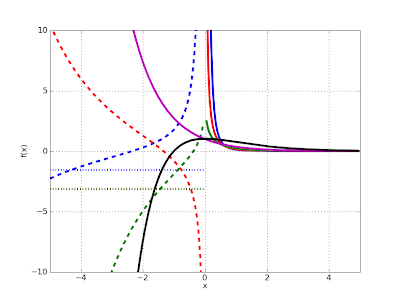`T1 = lambda x: expint(-2,x)T2 = lambda x: expint(-1,x)T3 = lambda x: expint(0,x)T4 = lambda x: expint(1,x)T5 = lambda x: expint(2,x)plot([T1,T2,T3,T4,T5],[-5,5],[-10,10])`And a complex plot of gammainc(3+4j, 1/z):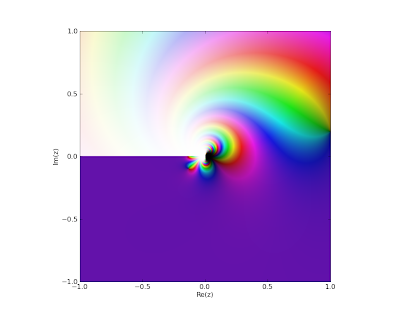A plot of gammainc(1/z, -1/z, 1/z); a rather nonsensical function (but that is besides the point):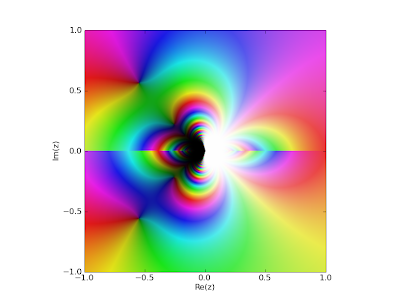#### Clausen functions

Unrelated to the gamma functions, I've also implemented Clausen functions: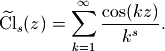These functions are just polylogarithms in disguise, but convenient as standalone functions. With them one can evaluate certain divergent Fourier series for example:

`>>> clsin(-2, 3)-0.01781786725924798006896962>>> nsum(lambda k: k**2 * sin(3*k), [1,inf])-0.01781786725924798006896962`

They also work for complex arguments (and are related to zeta functions):

`>>> clsin(2+3j, 1+2j)(1.352229437254898401329125 + 1.401881614736751520048876j)>>> clcos(2+3j, pi)(-1.042010539574581174661637 - 0.2070574989958949174656102j)>>> altzeta(2+3j)(1.042010539574581174661637 + 0.2070574989958949174656102j)>>> chop(clcos(zetazero(2), pi/2))0.0`